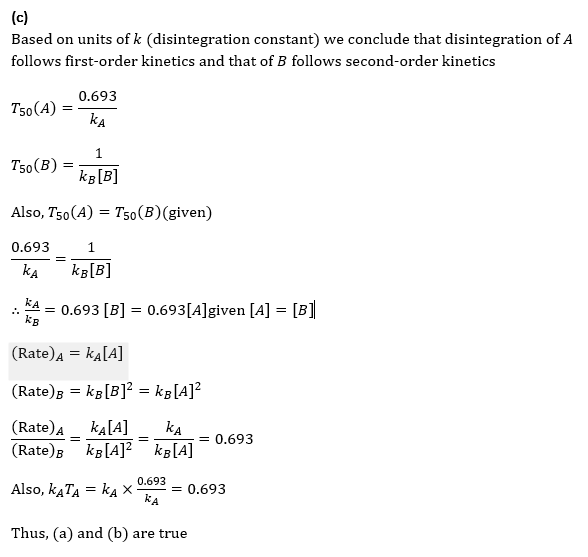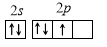## Chemistry Aptitude Quiz

IIT JEE exam which consists of JEE Main and JEE Advanced is one of the most important entrance exams for engineering aspirants. The exam is held for candidates who are aspiring to pursue a career in the field of engineering and technical studies. Chemistry is important because everything you do is chemistry! Even your body is made of chemicals. Chemical reactions occur when you breathe, eat, or just sit there reading. All matter is made of chemicals, so the importance of chemistry is that it's the study of everything..

Q1. Which is different in isotopes of an element?
•  Atomic number
•  Mass number
•  Number of protons
•  Number of electrons
Solution
(b) Isotopes have same atomic number but different mass number

Q2.If uranium (mass number 238 and atomic number 92) emits an α-particle, the product has mass number and atomic number
•  236 and 92
•  234 and 90
•  238 and 90
•  236 and 90
Solution
(b) Emission of an α-particle means mass is decreased by 4 units and charge by 2 units. Thus, _92 U^238 □(→┴(-α ) ) _90 U^234 Thus, the mass number = 234 Atomic number = 90

Q3.  Which of the following is false?
•  The angular momentum of an electron due to its spinning is given as √(s(s+1)) (h/2π), where s can take a value of 1/2
•  The angular momentum of an electron due to its spinning is given as m_s (h/2π), where m_s can take the value of +1⁄2
•  The azimuthal quantum number cannot have negative values
•  The potential energy of an electron in an orbit is twice in magnitude as compared to its kinetic energy
Solution
(b) True. False. The expression m_s (h/2π) is that of z-component of angular momentum True. The azimuthal quantum number has the value 0,1,2,…,(n-1) True. The expressions are KE=1/2 mv^2=1/2 (Ze^2)/(4πε_0 )r PE=-(Ze^2)/(4πε_0 )r
Q4.   A (→┴k_A )┬(T_A )productB (→┴k_B )┬(T_B ) product Aand Bare two radioactive elements with half-life periods T_Aand T_B(in years) and k_A (year^(-1) )and k_B (atom^(-1) year^(-1) ). If half-life periods are equal, disintegration rate at the start of disintegration with same concentration would be
•  k_A T_A
•  0.693
•  Both (a) and (b)
•  None of these
SolutionQ5.••••Solution
Part B

Q6. Two nuclei are not identical but have the same number of nucleons. These are
•  Isotopes
•  Isobars
• Isotones
•  None
Solution
Part B

Q7.When 〖 _17 Cl〗^35 undergoes (n,p) reaction, the radioisotope formed is
•  〖 _15 P〗^32
•  〖 _16 S〗^35
•  〖 _16 S〗^34
•  〖 _15 P〗^34
Solution
Part B

Q8.For an α-emitting isotope, the value of disintegration constant is 0.49×10^(-10)per year. The amount of the isotope of a given sample will reduce to half its value after a period (in years) of nearly
•  0.45×10^10
•  0.9×10^10
•  1.41×10^10
•  2.82×10^10
Solution
(c) Half-life =0.693/λ=0.693/(0.49×10^(-10) ) yr =1.41×10^10 yr

Q9.The number of spherical nodes in 3p orbital are:
•  One
•  Three
•  None
•  Two
Solution
(a) Angular nodes =1, Spherical nodes =n-l-1=3-1-1=1

Q10. The transition of electrons in H atom that will emit maximum energy is
•  n_3→n_2
•  n_4→n_3
•  n_5→n_4
• n_6→n_5
Solution
(a)
n_3→n_2#### Written by: AUTHORNAME

AUTHORDESCRIPTION## Want to know more

Please fill in the details below:

## Latest NEET Articles\$type=three\$c=3\$author=hide\$comment=hide\$rm=hide\$date=hide\$snippet=hide

Name

ltr
item
BEST NEET COACHING CENTER | BEST IIT JEE COACHING INSTITUTE | BEST NEET & IIT JEE COACHING: STRUCTURE OF ATOM Quiz-9
STRUCTURE OF ATOM Quiz-9
https://1.bp.blogspot.com/-rUzAvbm91pU/YOgSmWoHViI/AAAAAAAA39o/pfDKdEakQ8gQo9xXmzgT2ZaorpUZPH9wACLcBGAsYHQ/s960/Quiz%2BImage%2B20%2B%252833%2529.jpg
https://1.bp.blogspot.com/-rUzAvbm91pU/YOgSmWoHViI/AAAAAAAA39o/pfDKdEakQ8gQo9xXmzgT2ZaorpUZPH9wACLcBGAsYHQ/s72-c/Quiz%2BImage%2B20%2B%252833%2529.jpg
BEST NEET COACHING CENTER | BEST IIT JEE COACHING INSTITUTE | BEST NEET & IIT JEE COACHING
https://www.cleariitmedical.com/2021/07/structure-of-atom-quiz-9.html
https://www.cleariitmedical.com/
https://www.cleariitmedical.com/
https://www.cleariitmedical.com/2021/07/structure-of-atom-quiz-9.html
true
7783647550433378923
UTF-8

STAY CONNECTED